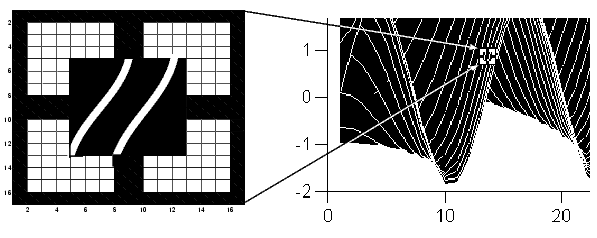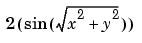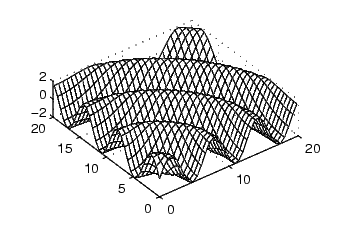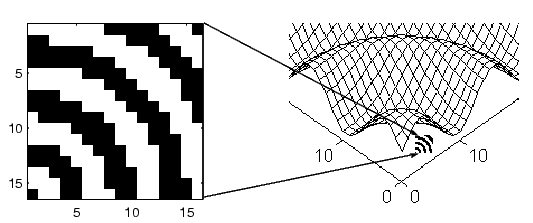GraphicsDefining Custom Pointers

When you set the `Pointer` property to `custom`, MATLAB displays the pointer you define using the `PointerShapeCData` and the `PointerShapeHotSpot` properties. Custom pointers are 16-by-16 pixels, where each pixel can be either black, white, or transparent.

Specify the pointer by creating a 16-by-16 matrix containing elements that are

• 1's where you want the pixel black
• 2's where you want the pixel white
• `NaN`s where you want the pixel transparent

Assign the matrix to the figure `PointerShapeCData` property. MATLAB displays the defined pointer whenever the pointer is in the figure window.

The `PointerShapeHotSpot` property specifies the pixel that indicates the pointer location. MATLAB then stores this location in the root `PointerLocation` property. Set the `PointerShapeHotSpot` property to a two-element vector specifying the row and column indices in the `PointerShapeCData` matrix that correspond to the pixel specifying the location. The default value for this property is [1 1], which corresponds to the upper left corner of the pointer.

Example -- Two Custom Pointers

One way to create a custom pointer is to assign values to a 16-by-16 matrix by hand, as illustrated in the following example.

First, initialize the matrix, setting all values to 2. Create a black border 1 pixel wide. Add alignment marks.

• ```P = ones(16)+1;
P(1,:) = 1; P(16,:) = 1;
P(:,1) = 1; P(:,16) = 1;
P(1:4,8:9) = 1; P(13:16,8:9) = 1;
P(8:9,1:4) = 1; P(8:9,13:16) = 1;
P(5:12,5:12) = NaN; ```% Create a transparent region in the center
```set(gcf,'Pointer','custom','PointerShapeCData',P,...
'PointerShapeHotSpot',[9 9])
```

The last statement sets the `Pointer` property to `custom`, assigns the matrix to the `PointerShapeCData` property, and selects element (9,9) as the "hot spot."

MATLAB now uses the custom pointer within the figure window.Creating Pointers from Functions.   You can use a mathematical function to define the `PointerShapeCData` matrix. For example, evaluating the function• ```g = 0:.2:20;
[X,Y] = meshgrid(g);
Z = 2*sin(sqrt(X.^2 + Y.^2));
mesh(Z);
```

produces an interesting surface.Use the values of `Z` to create a pointer sampling fewer points so that `Z` is a 16-by-16 matrix.

• ```g = linspace(0,20,16);
[X,Y] = meshgrid(g);
Z = 2*sin(sqrt(X.^2 + Y.^2));
set(gcf,'Pointer','custom',...
'PointerShapeCData',flipud((Z>0) + 1))
```

The statement `flipud((Z>0) + 1)` sets all values in `Z` that are greater than 0 to 2 (in MATLAB, true + 1 = 2), less than 0 to 1 (false + 1 = 1) and then flips the data around so that element (1,1) is the upper left corner.Specifying the Figure Pointer Axes Properties